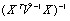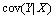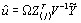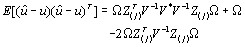# Sandwich estimators for standard errors

## Can MLwiN produce robust standard errors?

Sandwich estimators for standard errors are often useful, eg when model based estimators are very complex and difficult to compute and robust alternatives are required.

Consider the fixed part parameter estimatesThe covariance matrix is given byIf we replace the central covariance term by the usual (Normal) model based value, V, we obtain the usual formulawith sample estimates being substituted. The sandwich estimator is formed by replacing the estimate of the central covariance term,, by an empirical estimator based on the (block diagonal structure) cross product matrix, namely## Residuals

For residuals the estimated set of residuals for the j-th block at level h, using a similar notation to Goldstein (1995, App. 2.2) omitting the sub/superscript h, is given byTo obtain consistent estimators of the covariance matrix of these residuals (ignoring variation in the fixed parameter estimates) we can choose comparative or diagnostic estimators.

The diagnostic estimator is given byIf the model based estimatoris used this reduces to the expression given by Goldstein (1995, Appendix 2.2), otherwise the cross product matrix estimatoris used.

For the comparative estimator we havewhich reduces to the expression in Goldstein (1995, Appendix 2.2) when the model based estimatoris used.

In MLwiN 1.1 access to the sandwich estimators is via the FSDE and RSDE commands

For residuals, sandwich estimators will automatically be used when weighted residuals are specified in the residuals section on weighting for details of residuals produced from weighted models.